﻿ 广义度量空间问题成立的可能性 The Possibility of the Problem on Generalized Metric Space

Pure Mathematics
Vol. 09  No. 03 ( 2019 ), Article ID: 30292 , 8 pages
10.12677/PM.2019.93045

The Possibility of the Problem on Generalized Metric Space

—Space M1 Equivalent to Space M3

Jiaming Luo

School of Mathematics and Statistics, Henan University of Science and Technology, Luoyang Henan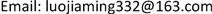Received: Apr. 24th, 2019; accepted: May 4th, 2019; published: May 20th, 2019ABSTRACT

This paper tries to prove the equivalence of stratifiable spaces in the problem on generalized metric space. The definitions and properties of finite basis and regularity in metric space are studied. The regular space with $\sigma$ local finite basis is obtained. In addition, the method of building space is obtained by using the Nagata-Smirnov Metrization Theorem to weaken the $\sigma$ local finite basis to the closure keep basis. A method of constructing a topological space and establishing a continuous mapping is used here. The space M1 is equal to the space M3. In this way, three kinds of spatial equivalent problems in generalized metric space are solved.

Keywords:Metric Space, Stratifiable Space, Regular Space, Open Set, Closure——M1空间等价于M3空间Copyright © 2019 by author(s) and Hans Publishers Inc.1. 引言

2. M1与M3等价的证明过程

2.1. 引入紧缩映射 $\Phi$$\Phi$ 具有如下性质

1) 若 $A\cap B=\varnothing$，显然 ${H}_{A}\cap {H}_{B}=\varnothing$

2) 若 $A\cap B\ne \varnothing$，必有 ${H}_{A}\cap {H}_{B}=\varnothing$

1)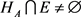${H}_{B}\cap E\ne \varnothing$

2)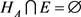${H}_{B}\cap E\ne \varnothing$

3) ${H}_{A}\cap E\ne \varnothing$${H}_{B}\cap E=\varnothing$

4) ${H}_{A}\cap E=\varnothing$${H}_{B}\cap E=\varnothing$

2.2. 紧缩映射的性质的进一步讨论

3. 层空间对应关系的讨论

3.1. 证明 ${H}_{{M}_{1}}$ 空间是 ${M}_{1}$ 空间

${T}_{11}=\underset{i=1}{\overset{\infty }{\cup }}{W}_{i11}\cup {A}_{1},\cdots ,{T}_{1n}=\underset{i=1}{\overset{\infty }{\cup }}{W}_{i1n}\cup {A}_{1};\cdots ;{T}_{nn}=\underset{i=1}{\overset{\infty }{\cup }}{W}_{inn}\cup {A}_{n}$.

$\underset{i,k}{\overset{\infty }{\cap }}{T}_{ik}={A}_{k}$ 时， $\left(\underset{i=1}{\overset{\infty }{\cap }}{T}_{i1}\right)\cup \left(\underset{i=1}{\overset{\infty }{\cap }}{T}_{i2}\right)\cup \cdots ={A}_{1}\cup {A}_{2},\cdots$ 显然成立。

${T}_{it}=\left\{{T}_{it}-\left({Q}_{t}\cup {A}_{t}\right)\right\}\cup \left({Q}_{t}\cup {A}_{t}\right)=\underset{g=1}{\overset{\infty }{\cup }}{K}_{g}\cup {A}_{t}$.

$\left(\underset{i=1}{\overset{\infty }{\cap }}{T}_{i1}\right)\cup \left(\underset{i=1}{\overset{\infty }{\cap }}{T}_{i2}\right)\cup \cdots =\left(\underset{e=1}{\overset{\infty }{\cup }}{Q}_{e}\right)\cup \left(\underset{e=1}{\overset{\infty }{\cup }}{A}_{e}\right)=\underset{j=1}{\overset{\infty }{\cap }}{N}_{j}=\underset{\mu =1}{\overset{\infty }{\cup }}{A}_{\mu }\cup P$

${N}_{j}\cap {T}_{i1}={A}_{1},{N}_{j}\cap {T}_{i2}={A}_{2},\cdots$，其中$⇒\underset{i,j,\Sigma }{\overset{\infty }{\cup }}\left({N}_{j}\cap {T}_{i\Sigma }\right)=\underset{\varphi =1}{\overset{\infty }{\cup }}{A}_{\varphi }⇒\left(\underset{j=1}{\overset{\infty }{\cup }}{N}_{j}\right)\cap \left(\underset{i,\Sigma }{\overset{\infty }{\cup }}{T}_{i\Sigma }\right)=\underset{\varphi =1}{\overset{\infty }{\cup }}{A}_{\varphi }$.

$\left(\underset{i=1}{\overset{\infty }{\cap }}{T}_{i1}\right)\cup \left(\underset{i=1}{\overset{\infty }{\cap }}{T}_{i2}\right)\cup \cdots =\left(\underset{e=1}{\overset{\infty }{\cup }}{Q}_{e}\right)\cup \left(\underset{e=1}{\overset{\infty }{\cup }}{A}_{e}\right)$ ,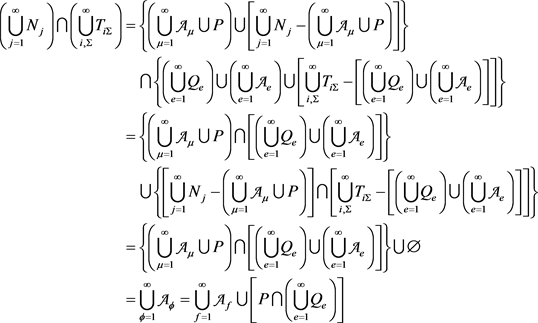, (1)

$\left\{{P}_{i}-\left(\underset{\varphi =1}{\overset{\infty }{\cup }}{A}_{\varphi }\right)\right\}\cap \left\{\left(\underset{e=1}{\overset{\infty }{\cup }}{Q}_{e}\right)-\left(\underset{\varphi =1}{\overset{\infty }{\cup }}{A}_{\varphi }\right)\right\}\subset \underset{\varphi =1}{\overset{\infty }{\cup }}{A}_{\varphi }$，同样地，也可能出现例如 $\underset{\varphi =1}{\overset{\infty }{\cup }}{A}_{\varphi }\subset P$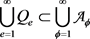，如此则总有 $\underset{f=1}{\overset{\infty }{\cup }}{A}_{f}\cup \left\{P-\left(\underset{\varphi =1}{\overset{\infty }{\cup }}{A}_{\varphi }\right)\right\}=\underset{\varphi =1}{\overset{\infty }{\cup }}{A}_{\varphi }⇒P-\left(\underset{\varphi =1}{\overset{\infty }{\cup }}{A}_{\varphi }\right)=\varnothing ⇒P\subset \underset{\varphi =1}{\overset{\infty }{\cup }}{A}_{\varphi }$

${N}_{j}\cap {T}_{i1}={A}_{1}\cup {B}_{1},{N}_{j}\cap {T}_{i2}={A}_{2}\cup {B}_{2},\cdots$ 时，可由(1)式得到

$\left(\underset{j=1}{\overset{\infty }{\cup }}{N}_{j}\right)\cap \left(\underset{i,\Sigma }{\overset{\infty }{\cup }}{T}_{i\Sigma }\right)=\underset{f=1}{\overset{\infty }{\cup }}{A}_{f}\cup \left[P\cap \left(\underset{e=1}{\overset{\infty }{\cup }}{Q}_{e}\right)\right]=\left(\underset{\varphi =1}{\overset{\infty }{\cup }}{A}_{\varphi }\right)\cup \left(\underset{\varphi =1}{\overset{\infty }{\cup }}{B}_{\varphi }\right)$ ,

${N}_{j}\cap {T}_{i1}={T}_{i1},{N}_{j}\cap {T}_{i2}={T}_{i2},\cdots$ 时，仍然由(1)式可得：

$\left(\underset{j=1}{\overset{\infty }{\cup }}{N}_{j}\right)\cap \left(\underset{i,\Sigma }{\overset{\infty }{\cup }}{T}_{i\Sigma }\right)=\underset{f=1}{\overset{\infty }{\cup }}{A}_{f}\cup \left[P\cap \left(\underset{e=1}{\overset{\infty }{\cup }}{Q}_{e}\right)\right]=\underset{i,\Sigma }{\overset{\infty }{\cup }}{T}_{i\Sigma }$ ;

$P\cap \left(\underset{e=1}{\overset{\infty }{\cup }}{Q}_{e}\right)=\varnothing$ 时，即 $\underset{f=1}{\overset{\infty }{\cup }}{A}_{f}\cup \varnothing =\underset{f=1}{\overset{\infty }{\cup }}{A}_{f}=\underset{i,\Sigma }{\overset{\infty }{\cup }}{T}_{i\Sigma }$ 与之前所假设情况的其中之一符合，所以显然成立；当 $P\cap \left(\underset{e=1}{\overset{\infty }{\cup }}{Q}_{e}\right)=Z\ne \varnothing$ 时，得 $\underset{f=1}{\overset{\infty }{\cup }}{A}_{f}\cup Z=\underset{i,\Sigma }{\overset{\infty }{\cup }}{T}_{i\Sigma }$ 也同样如此。因此不论 $P\cap \left(\underset{e=1}{\overset{\infty }{\cup }}{Q}_{e}\right)$ 的情况如何，都有 $P=\underset{e=1}{\overset{\infty }{\cup }}{Q}_{e}$ 成立。所以式子 $\left(\underset{i=1}{\overset{\infty }{\cap }}{T}_{i1}\right)\cup \left(\underset{i=1}{\overset{\infty }{\cap }}{T}_{i2}\right)\cup \cdots =\underset{j=1}{\overset{\infty }{\cap }}{N}_{j}$ 得证。所以经映射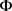作用后所得到的空间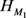的确是 ${M}_{1}$ 空间。

3.2. 证明 ${H}_{{M}_{3}}$ 空间是 ${M}_{3}$ 空间

${E}_{1}\subset {U}_{11},{U}_{21},\cdots ;\cdots ;{E}_{n}\subset {U}_{1n},{U}_{2n},\cdots$.

${M}_{3}$ 空间的定义可类似地对 ${H}_{M3}$ 就有，对于另一闭集D， ${G}_{1},{G}_{2},\cdots$ 为开集，所以可设

${E}_{1}\cup {{E}^{\prime }}_{1}=\underset{k=1}{\overset{\infty }{\cap }}{U}_{K1},{E}_{2}\cup {{E}^{\prime }}_{2}\subset \underset{K=1}{\overset{\infty }{\cap }}{U}_{K2},\cdots$.

$\begin{array}{c}C=\left({E}_{1}\cup {{E}^{\prime }}_{1}\right)\cap \left({E}_{2}\cup {{E}^{\prime }}_{2}\right)\cap \cdots =\left({E}_{1}\cap {E}_{2}\cap \cdots \right)\cup \left({{E}^{\prime }}_{1}\cap {{E}^{\prime }}_{2}\cap \cdots \right)\\ =\left(\underset{c=1}{\overset{\infty }{\cap }}{E}_{c}\right)\cup \left(\underset{d=1}{\overset{\infty }{\cap }}{{E}^{\prime }}_{d}\right)={C}^{\prime }\cup \left(\underset{d=1}{\overset{\infty }{\cap }}{{E}^{\prime }}_{d}\right)\end{array}$ ,

$D=\left({G}_{1}\cup {{G}^{\prime }}_{1}\right)\cap \left({G}_{2}\cup {{G}^{\prime }}_{2}\right)\cap \cdots =\left(\underset{a=1}{\overset{\infty }{\cap }}{G}_{a}\right)\cup \left(\underset{b=1}{\overset{\infty }{\cap }}{{G}^{\prime }}_{b}\right)={D}^{\prime }\cup \left(\underset{b=1}{\overset{\infty }{\cap }}{{G}^{\prime }}_{b}\right)$

$C=D$ 时，就有 $D-\left(\underset{b=1}{\overset{\infty }{\cap }}{{G}^{\prime }}_{b}\right)=C-\left(\underset{d=1}{\overset{\infty }{\cap }}{{E}^{\prime }}_{d}\right)$，当 $\left({E}_{i}-C\right)\cup \left(\underset{d=1}{\overset{\infty }{\cap }}{{E}^{\prime }}_{d}\right)\subset \left({G}_{j}-D\right)\cup \left(\underset{b=1}{\overset{\infty }{\cap }}{{G}^{\prime }}_{b}\right)$ 时， ${H}_{{M}_{3}}$ 是广义度量空间。

$C\subset D$ 时，设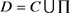，所以 $D-\left(\underset{b=1}{\overset{\infty }{\cap }}{{G}^{\prime }}_{b}\right)=\left[C-\left(\underset{d=1}{\overset{\infty }{\cap }}{{E}^{\prime }}_{d}\right)\right]\cup \prod$，所以当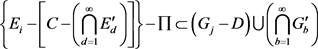$\left({E}_{i}-C\right)\cup \left(\underset{d=1}{\overset{\infty }{\cap }}{{E}^{\prime }}_{d}\right)\subset \left({G}_{j}-D\right)\cup \left(\underset{b=1}{\overset{\infty }{\cap }}{{G}^{\prime }}_{b}\right)\cup \prod$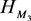是广义度量空间。

$C,D$ 为闭集， ${E}_{1},{E}_{2},\cdots$${G}_{1},{G}_{2},\cdots$ 为开集并且

${E}_{1}\subset \underset{k=1}{\overset{\infty }{\cap }}{U}_{k1},{E}_{2}\subset \underset{k=1}{\overset{\infty }{\cap }}{U}_{k2},\cdots$ , ${G}_{1}\subset \underset{\delta =1}{\overset{\infty }{\cap }}{F}_{\delta 1},{G}_{2}\subset \underset{\delta =1}{\overset{\infty }{\cap }}{F}_{\delta 2},\cdots$.

$D=\left({G}_{1}\cup {L}_{1}\right)\cap \left({G}_{2}\cup {L}_{2}\right)\cap \cdots =\left(\underset{\delta =1}{\overset{\infty }{\cap }}{G}_{\delta }\right)\cup \left(\underset{\lambda =1}{\overset{\infty }{\cap }}{L}_{\lambda }\right)$

$C=\left({E}_{1}\cup {K}_{1}\right)\cap \left({E}_{2}\cup {K}_{2}\right)\cap \cdots =\left(\underset{k=1}{\overset{\infty }{\cap }}{E}_{k}\right)\cup \left(\underset{\mu =1}{\overset{\infty }{\cap }}{K}_{\mu }\right)$.

$D=\left(\underset{k=1}{\overset{\infty }{\cap }}{E}_{k}\right)\cup \left(\underset{s=1}{\overset{\infty }{\cap }}{J}_{s}\right)\cup \left(\underset{\lambda =1}{\overset{\infty }{\cap }}{L}_{\lambda }\right)=\left\{C-\left(\underset{\mu =1}{\overset{\infty }{\cap }}{K}_{\mu }\right)\right\}\cup \left(\underset{s=1}{\overset{\infty }{\cap }}{J}_{s}\right)\cup \left(\underset{\lambda =1}{\overset{\infty }{\cap }}{L}_{\lambda }\right)$.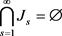$\underset{\mu =1}{\overset{\infty }{\cap }}{K}_{\mu }\subset \underset{\lambda =1}{\overset{\infty }{\cap }}{L}_{\lambda }$ 时有 $C\subset D$ 成立。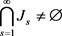时有 $C\subset D$ 成立。

3.3. 讨论 ${H}_{{M}_{1}}\sim {H}_{{M}_{3}}$ 的成立性

$C,E\subset A\subset {H}_{{M}_{1}}$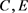都是闭集， $A$ 为保持基，若 $E=\left(\underset{t=1}{\overset{\infty }{\cup }}{W}_{t}\right)\cap C$，其中 ${W}_{1},{W}_{2},\cdots$$C,E$ 都是闭集，且E满足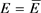，令 $C={J}_{1}\cup {J}_{2}\cup \cdots$。因为由保持基的条件就有

$\left(\underset{i=1}{\overset{\infty }{\cap }}{R}_{i1}\right)\cup \left(\underset{i=1}{\overset{\infty }{\cap }}{R}_{i2}\right)\cup \cdots =\underset{j=1}{\overset{\infty }{\cap }}{S}_{j}$ (2)

$\left\{\left(\underset{i=1}{\overset{\infty }{\cap }}{R}_{i1}\right)\cup \left(\underset{i=1}{\overset{\infty }{\cap }}{R}_{i2}\right)\cup \cdots \right\}\cap \left\{\left(\underset{i=1}{\overset{\infty }{\cap }}{K}_{i1}\right)\cup \left(\underset{i=1}{\overset{\infty }{\cap }}{K}_{i2}\right)\cup \cdots \right\}=\left(\underset{j=1}{\overset{\infty }{\cap }}{S}_{j}\right)\cap \left(\underset{j=1}{\overset{\infty }{\cap }}{L}_{j}\right)=\underset{\theta =1}{\overset{\infty }{\cap }}\left\{\left(\underset{i=1}{\overset{\infty }{\cap }}{R}_{i\theta }\right)\cap \left(\underset{i=1}{\overset{\infty }{\cap }}{K}_{i\theta }\right)\right\}$

${J}_{1}\cup {Z}_{1}=\underset{i=1}{\overset{\infty }{\cap }}{K}_{i1},{J}_{2}\cup {Z}_{2}=\underset{i=1}{\overset{\infty }{\cap }}{K}_{i2},\cdots$ ,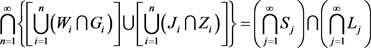. (4)

$\underset{i=1}{\overset{\infty }{\cap }}{T}_{i1}={P}_{1}\cup {Q}_{1},\underset{i=1}{\overset{\infty }{\cap }}{T}_{i2}={P}_{2}\cup {Q}_{2},\cdots$

$\left(\underset{i=1}{\overset{\infty }{\cap }}{T}_{i1}\right)\cap \left(\underset{i=1}{\overset{\infty }{\cap }}{T}_{i2}\right)\cap \cdots =E$ ,

$C=\left(\underset{\epsilon =1}{\overset{\infty }{\cap }}{I}_{\epsilon 1}\right)\cap \left(\underset{\epsilon =1}{\overset{\infty }{\cap }}{I}_{\epsilon 2}\right)\cap \cdots =\left(\underset{c=1}{\overset{\infty }{\cap }}{F}_{c}\right)\cup \left(\underset{d=1}{\overset{\infty }{\cap }}{{F}^{\prime }}_{d}\right)$，所以 $\left(\underset{c=1}{\overset{\infty }{\cap }}{F}_{c}\right)\cup \left(\underset{d=1}{\overset{\infty }{\cap }}{{F}^{\prime }}_{d}\right)\cup Q=\left(\underset{a=1}{\overset{\infty }{\cap }}{E}_{a}\right)\cup \left(\underset{b=1}{\overset{\infty }{\cap }}{{E}^{\prime }}_{b}\right)$

$C,D\subset {H}_{{M}_{3}}$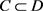$C,D$ 都是闭集，若 $C=D=\underset{t=1}{\overset{\infty }{\cup }}{V}_{t}$，且有一闭集可表示为开集的闭包的交，即有 $C=\underset{k,l}{\overset{\infty }{\cap }}{U}_{kl}=D=\underset{t=1}{\overset{\infty }{\cup }}{V}_{t}$。当 ${V}_{1}=\underset{i=1}{\overset{\infty }{\cap }}{E}_{i1},{V}_{2}=\underset{i=1}{\overset{\infty }{\cap }}{E}_{i2},\cdots$ 时， ${E}_{i1}$${V}_{1}={W}_{11}\cup {W}_{21}\cup \cdots$${W}_{i1}$ 的闭包，……， ${E}_{in}$${V}_{n}={W}_{1n}\cup {W}_{2n}\cup \cdots$${W}_{in}$ 的闭包，……。所以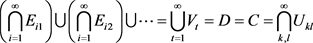,

$C\subset D$，设 $D=C\cup Q=\underset{t=1}{\overset{\infty }{\cup }}{V}_{t}\cup Q$$C,D$ 是闭集，且有 $D=\underset{\delta ,\theta }{\overset{\infty }{\cap }}{G}_{\delta \theta }$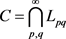。因为 $G\left(n,C\right)\subset G\left(n,D\right)$，所以有 ${M}_{1}\subset \underset{\delta =1}{\overset{\infty }{\cap }}{G}_{\delta 1},{M}_{2}\subset \underset{\delta =1}{\overset{\infty }{\cap }}{G}_{\delta 2},\cdots$${N}_{1}\subset \underset{p=1}{\overset{\infty }{\cap }}{L}_{p1},{N}_{2}\subset \underset{p=1}{\overset{\infty }{\cap }}{L}_{p2},\cdots$ 所以设 ${N}_{i}\cup P={M}_{j}$ 其中，同样也有 ${M}_{1}\cup {{M}^{\prime }}_{1}=\underset{\delta =1}{\overset{\infty }{\cap }}{G}_{\delta 1},{M}_{2}\cup {{M}^{\prime }}_{2}=\underset{\delta =1}{\overset{\infty }{\cap }}{G}_{\delta 2},\cdots$${N}_{1}\cup {{N}^{\prime }}_{1}=\underset{p=1}{\overset{\infty }{\cap }}{L}_{p1},{N}_{2}\cup {{N}^{\prime }}_{2}=\underset{p=1}{\overset{\infty }{\cap }}{L}_{p2},\cdots$ 所以

$D=\underset{\delta ,\theta }{\overset{\infty }{\cap }}{G}_{\delta \theta }=\left({M}_{1}\cup {{M}^{\prime }}_{1}\right)\cap \left({M}_{2}\cup {{M}^{\prime }}_{2}\right)\cap \cdots =\left(\underset{a=1}{\overset{\infty }{\cap }}{M}_{a}\right)\cup \left(\underset{b=1}{\overset{\infty }{\cap }}{{M}^{\prime }}_{b}\right)=\left(\underset{a=1}{\overset{\infty }{\cap }}{N}_{a}\right)\cup \left(\underset{\epsilon =1}{\overset{\infty }{\cap }}{P}_{\epsilon }\right)\cup \left(\underset{b=1}{\overset{\infty }{\cap }}{{M}^{\prime }}_{b}\right)$

$C=\underset{p,q}{\overset{\infty }{\cap }}{L}_{pq}=\left({N}_{1}\cup {{N}^{\prime }}_{1}\right)\cap \left({N}_{2}\cup {{N}^{\prime }}_{2}\right)\cap \cdots =\left(\underset{c=1}{\overset{\infty }{\cap }}{N}_{c}\right)\cup \left(\underset{d=1}{\overset{\infty }{\cap }}{{N}^{\prime }}_{d}\right)$

$Q=D-C=\left\{\left(\underset{\epsilon =1}{\overset{\infty }{\cap }}{P}_{\epsilon }\right)\cup \left(\underset{b=1}{\overset{\infty }{\cap }}{{M}^{\prime }}_{b}\right)\right\}-\left(\underset{d=1}{\overset{\infty }{\cap }}{{N}^{\prime }}_{d}\right)\ne \varnothing$.

4. 总结

The Possibility of the Problem on Generalized Metric Space[J]. 理论数学, 2019, 09(03): 336-343. https://doi.org/10.12677/PM.2019.93045

1. 1. “10000个科学难题”数学编委会. 10000个科学难题•数学卷[M]. 北京: 科学出版社, 2009: 211-212.

2. 2. James R. Munkres. 拓扑学[M]. 北京: 机械工业出版社, 2006.

3. 3. 儿玉之宏, 永见启应. 拓扑空间论[M]. 北京: 科学出版社, 1984.

4. 4. 高国士. 拓扑空间论[M]. 北京: 科学出版社, 2000.

5. 5. Anderson, R.D., et al. (2002) Recent Progress in General Topology. North-Holland, Amsterdam, 545-575.

6. 6. Gartside, P.M. and Reznichenko, E.A. (2000) Near Metric Properties of Function Spaces. Fundamenta Mathematicae, 164, 97-114.